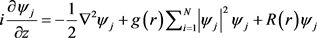# 二维矢量多极孤子和涡旋孤子探究Research on Two-Dimensional Vector Multipole Solitons and Vortex Solitons

• 全文下载: PDF(743KB)    PP.191-195   DOI: 10.12677/MP.2019.94021
• 下载量: 286  浏览量: 442

In this paper, two-dimensional coupled nonlinear Schrödinger equations with spatial nonlinear modulation and lateral modulation are studied, and vector multipole and vortex soliton solutions are derived and analyzed. When the modulation depth is selected to be 0 and 1, the vector multi-pole and vortex soliton structures are obtained, respectively. The number of azimuthal lobes (the “petal" of a plurality of polarized solitons) is determined by the topological index m, and the number of layers in the multipole soliton is determined by the value of n.

1. 研究背景

2. 理论模型及形变约化(1)

${\psi }_{j}\left(r,\phi ,z\right)=A\left(r\right){\Phi }_{j}\left(\phi \right)\mathrm{exp}\left(-i\kappa z\right)$ (2)

$\frac{{r}^{2}}{A}\left\{\frac{{\partial }^{2}A}{\partial {r}^{2}}+\frac{1}{r}\frac{\partial A}{\partial r}+2\left[\kappa -R\left(r\right)\right]A-2g\left(r\right){A}^{3}\right\}={m}^{2}$ (3)

$-\frac{1}{{\varphi }_{j}}\frac{{\partial }^{2}{\varphi }_{j}}{\partial {\phi }^{2}}={m}^{2}$ (4)

${\Phi }_{j}={C}_{j}\mathrm{cos}\left(m\phi \right)+{D}_{j}\mathrm{sin}\left(m\phi \right)$ (5)

$A\left(r\right)=\rho \left(r\right)U\left[\chi \left(r\right)\right]$ 代入方程(3)，且 $U\left[\chi \left(r\right)\right]$ 满足

$-\frac{{\text{d}}^{2}U}{\text{d}{\chi }^{2}}+G\left(U\right)=\eta U$ (6)

${\rho }_{rr}+\frac{1}{r}{\rho }_{r}+\left[2\kappa -2R\left(r\right)-\frac{{m}^{2}}{{r}^{2}}\right]\rho =\frac{\eta }{{r}^{2}{\rho }^{3}}$ (7)

$\frac{G\left(U\right){\chi }_{r}^{2}}{{U}^{3}}-2g{\rho }^{2}=0$ (8)

$g\left(r\right)=G\left(U\right){r}^{-2}{\rho }^{-6}\left(r\right)/\left(2{U}^{3}\right)$$\chi \left(r\right)={\int }_{0}^{r}{\rho }^{-2}\left(s\right){s}^{-1}\text{d}s$ (9)

$\rho ={r}^{-1}\left[{c}_{1}M\left(\frac{\kappa }{2\sqrt{2\omega }},\frac{m}{2},\sqrt{2\omega }{r}^{2}\right)+{c}_{2}W\left(\frac{\kappa }{2\sqrt{2\omega }},\frac{m}{2},\sqrt{2\omega }{r}^{2}\right)\right]$ (10)

$\rho ={c}_{3}J\left(m,\sqrt{2\kappa }r\right)+{c}_{4}Y\left(m,\sqrt{2\kappa }r\right)$ (11)

$\rho =\sqrt{\frac{1}{r}\left(\alpha {\varphi }_{1}^{2}+2\beta {\varphi }_{1}{\varphi }_{2}+\gamma {\varphi }_{2}^{2}\right)}$ (12)

${\varphi }_{rr}+\left[2\kappa -2R\left(r\right)-\frac{{m}^{2}}{{r}^{2}}\right]\varphi =0$

$U\left(\chi \right)=\frac{2n\lambda }{\sqrt{-{g}_{0}}}sd\left[2n\lambda \chi \left(r\right),\frac{\sqrt{2}}{2}\right]$ (13)

3. 矢量多极孤子和涡旋孤子结构

4. 小结

  Quiroga-Teixeiro, M. and Michinel, H.J. (1997) Stable Azimuthal Stationary State in Quintic Nonlinear Optical Media. Journal of the Optical Society of America B, 14, 2004-2009. https://doi.org/10.1364/JOSAB.14.002004  Manakov, S.V. (1974) On the Theory of Two-Dimensional Sta-tionary Self-Focusing of Electromagnetic Waves. Soviet Physics—JETP, 38, 248-253.  Radhakrishnan, R. and Aravinthan, K.J. (2007) A Dark-Bright Optical Soliton Solution to the Coupled Nonlinear Schrödinger Equation. Journal of Physics A: Mathematical and Theoretical, 40, Article ID: 13023. https://doi.org/10.1088/1751-8113/40/43/011  Sun, Z.Y., Gao, Y.T., Yu, X., Liu, W.J. and Liu, Y. (2009) Bound Vector Solitons and Soliton Complexes for the Coupled Nonlinear Schrödinger Equations. Physical Review E, 80, Article ID: 066608. https://doi.org/10.1103/PhysRevE.80.066608  Desyatnikov, A.S., Kivshar, Y.S. and Torner, L. (2005) Optical Vortices and Vortex Solitons. Progress in Optics, 47, 291-391. https://doi.org/10.1016/S0079-6638(05)47006-7  Borovkova, O.V., Kartashov, Y.V., Torner, L. and Malomed, B.A. (2011) Bright Solitons from Defocusing Nonlinearities. Physical Review E, 84, Article ID: 035602(R). https://doi.org/10.1103/PhysRevE.84.035602  Zhong, W.P., Belic, M.R., Assanto, G., Malomed, B.A. and Huang, T.W. (2011) Self-Trapping of Scalar and Vector Dipole Solitary Waves in Kerr Media. Physical Review A, 83, Article ID: 043833. https://doi.org/10.1103/PhysRevA.83.043833